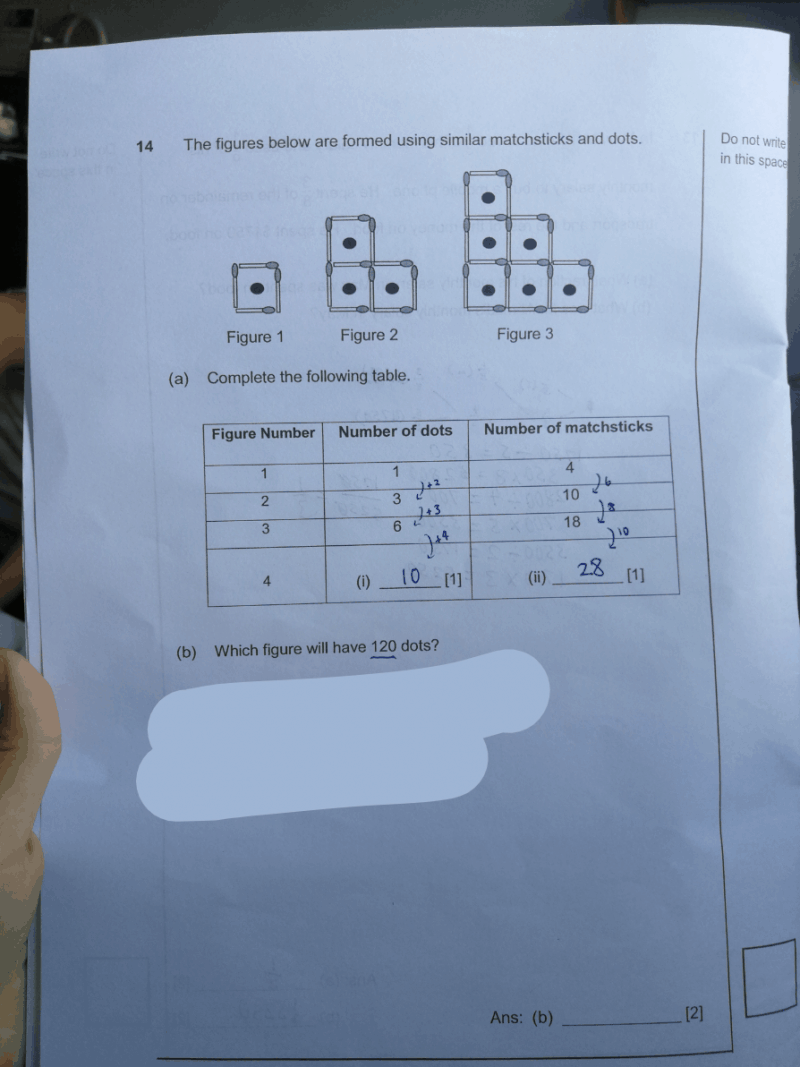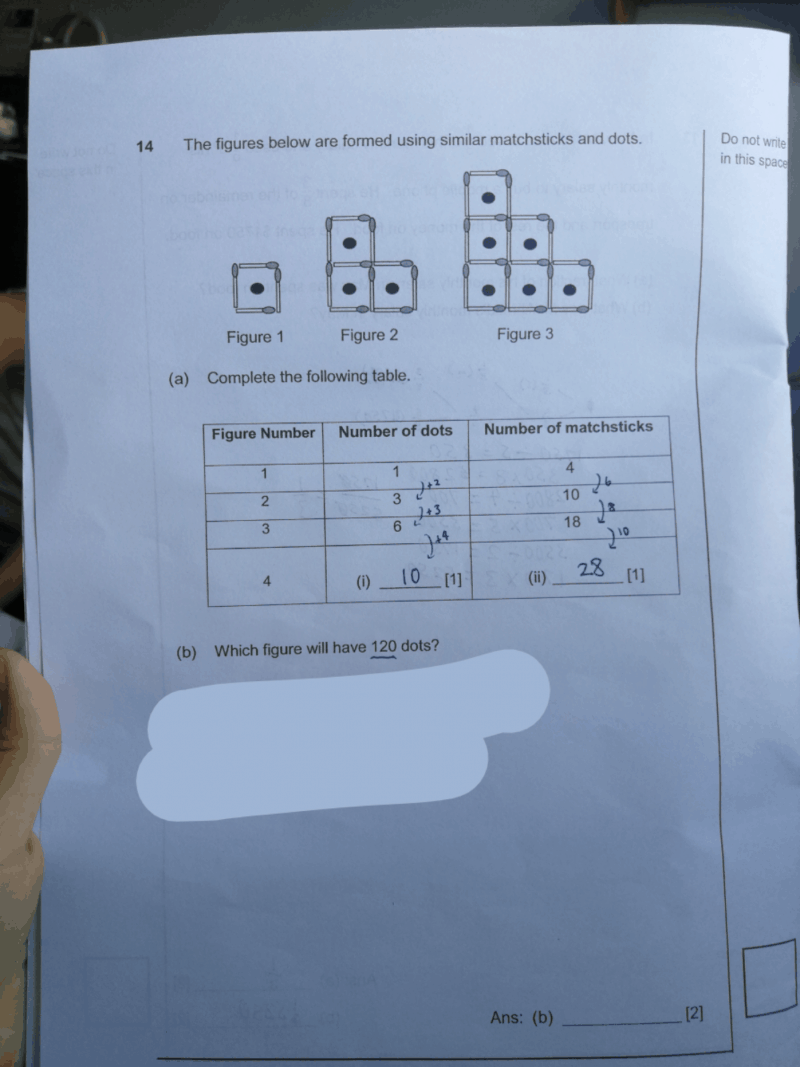# QuestionPls help

 Figure Number Number of dots 1 1 2 1 + 2 3 1 + 2 + 3 4 1 + 2 + 3 + 4 n 1 + 2 + 3 + 4 + … + n

It is good for your child to memorise that formula for 1 + 2 + 3 + 4 + … + n is

n(n + 1) ÷ 2

n(n + 1) ÷ 2 = 120

n(n + 1) = 240

n² + n = 240

Now, √240 = 15.4919…., so the answer should be 15.

Test

15 × 16 = 240

240 ÷ 2 = 120

Therefore, figure 15 has 120 dots.

0 Replies 1 Like ✔Accepted AnswerThere is a pattern for this. The figure number is equal to the number of dots in the largest diagonal row.

I used a calculator for this because i did additions to reach 120. I found the answer to be 15 in that way.

There may be other methods, but for me, I used this method which suits me best.

0 Replies 1 Like